# C++对象模型

+关注继续查看

——谈VC++对象模型
（美）简.格雷

1 前言

1* 类如何布局？
2* 成员变量如何访问？
3* 成员函数如何访问？
5* 使用如下机制时，开销如何：
* 单继承、多重继承、虚继承
* 虚函数调用
* 强制转换到基类，或者强制转换到虚基类
* 异常处理

2 类布局

2.1 C结构（struct）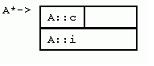1. struct  A {
2.    char  c;
3.    int  i;
4. };
[cpp] view plain
1. struct A {
2.    char c;
3.    int i;
4. };

2.2 有C++特征的C结构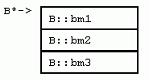1. struct  B {
2. public :
3.    int  bm1;
4. protected :
5.    int  bm2;
6. private :
7.    int  bm3;
8.    static   int  bsm;
9.    void  bf();
10.    static   void  bsf();
11.    typedef   void * bpv;
12.    struct  N { };
13. };
[cpp] view plain
1. struct B {
2. public:
3.    int bm1;
4. protected:
5.    int bm2;
6. private:
7.    int bm3;
8.    static int bsm;
9.    void bf();
10.    static void bsf();
11.    typedef void* bpv;
12.    struct N { };
13. };

2.3 单继承

C++ 提供继承的目的是在不同的类型之间提取共性。比如，科学家对物种进行分类，从而有种、属、纲等说法。有了这种层次结构，我们才可能将某些具备特定性质的东 西归入到最合适的分类层次上，如“怀孩子的是哺乳动物”。由于这些属性可以被子类继承，所以，我们只要知道“鲸鱼、人”是哺乳动物，就可以方便地指出“鲸 鱼、人都可以怀孩子”。那些特例，如鸭嘴兽（生蛋的哺乳动物），则要求我们对缺省的属性或行为进行覆盖。
C++中的继承语法很简单，在子类后加上“:base”就可以了。下面的D继承自基类C。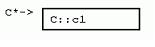1. struct  C {
2.    int  c1;
3.    void  cf();
4. };
[cpp] view plain
1. struct C {
2.    int c1;
3.    void cf();
4. };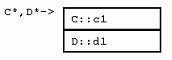1. struct  D : C {
2.    int  d1;
3.    void  df();
4. };
[cpp] view plain
1. struct D : C {
2.    int d1;
3.    void df();
4. };

2.4 多重继承

C++就允许用多重继承来解决这样的问题：

1. struct  Manager ... { ... };
2. struct  Worker ... { ... };
3. struct  MiddleManager : Manager, Worker { ... };
[cpp] view plain
1. struct Manager ... { ... };
2. struct Worker ... { ... };
3. struct MiddleManager : Manager, Worker { ... };1. struct  E {
2.    int  e1;
3.    void  ef();
4. };
[cpp] view plain
1. struct E {
2.    int e1;
3.    void ef();
4. };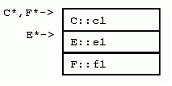1. struct  F : C, E {
2.    int  f1;
3.    void  ff();
4. };
[cpp] view plain
1. struct F : C, E {
2.    int f1;
3.    void ff();
4. };

1. F f;
2. // (void*)&f == (void*)(C*)&f;
3. // (void*)&f < (void*)(E*)&f;
[cpp] view plain
1. F f;
2. // (void*)&f == (void*)(C*)&f;
3. // (void*)&f < (void*)(E*)&f;

2.5 虚继承

1. struct  Employee { ... };
2. struct  Manager : Employee { ... };
3. struct  Worker : Employee { ... };
4. struct  MiddleManager : Manager, Worker { ... };
[cpp] view plain
1. struct Employee { ... };
2. struct Manager : Employee { ... };
3. struct Worker : Employee { ... };
4. struct MiddleManager : Manager, Worker { ... };

1. struct  Employee { ... };
2. struct  Manager :  virtual  Employee { ... };
3. struct  Worker :  virtual  Employee { ... };
4. struct  MiddleManager : Manager, Worker { ... };
[cpp] view plain
1. struct Employee { ... };
2. struct Manager : virtual Employee { ... };
3. struct Worker : virtual Employee { ... };
4. struct MiddleManager : Manager, Worker { ... };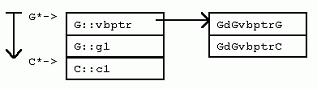1. struct  G :  virtual  C {
2.    int  g1;
3.    void  gf();
4. };
[c-sharp] view plain
1. struct G : virtual C {
2.    int g1;
3.    void gf();
4. };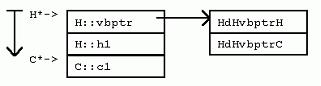1. struct  H :  virtual  C {
2.    int  h1;
3.    void  hf();
4. };
[cpp] view plain
1. struct H : virtual C {
2.    int h1;
3.    void hf();
4. };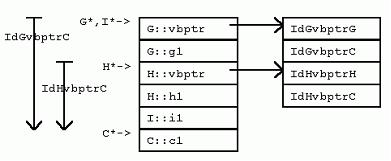1. struct  I : G, H {
2.    int  i1;
3.    void  _if();
4. };
[cpp] view plain
1. struct I : G, H {
2.    int i1;
3.    void _if();
4. };

VC++ 中，对每个继承自虚基类的类实例，将增加一个隐藏的“虚基类表指针”（vbptr） 成员变量，从而达到间接计算虚基类位置的目的。该变量指向一个全类共享的偏移量表，表中项目记录了对于该类 而言，“虚基类表指针”与虚基类之间的偏移量。

1 首先排列非虚继承的基类实例；
2 有虚基类时，为每个基类增加一个隐藏的vbptr，除非已经从非虚继承的类那里继承了一个vbptr；
3 排列派生类的新数据成员；
4 在实例最后，排列每个虚基类的一个实例。

3 成员变量

1. C* pc;
2. pc->c1; // *(pc + dCc1);
[cpp] view plain
1. C* pc;
2. pc->c1; // *(pc + dCc1);

a. 访问C的成员变量c1，只需要在pc上加上固定的偏移量dCc1（在C中，C指针地址与其c1成员变量之间的偏移量值），再获取该指针的内容即可。

1. D* pd;
2. pd->c1; // *(pd + dDC + dCc1); // *(pd + dDc1);
3. pd->d1; // *(pd + dDd1);
[cpp] view plain
1. D* pd;
2. pd->c1; // *(pd + dDC + dCc1); // *(pd + dDc1);
3. pd->d1; // *(pd + dDd1);

a. 当访问基类成员c1时，计算步骤本来应该为“pd+dDC+dCc1”，即为先计算D对象和C对象之间的偏移，再在此基础上加上C对象指针与成员变量c1 之间的偏移量。然而，由于dDC恒定为0，所以直接计算C对象地址与c1之间的偏移就可以了。
b. 当访问派生类成员d1时，直接计算偏移量。

1. F* pf;
2. pf->c1; // *(pf + dFC + dCc1); // *(pf + dFc1);
3. pf->e1; // *(pf + dFE + dEe1); // *(pf + dFe1);
4. pf->f1; // *(pf + dFf1);
[cpp] view plain
1. F* pf;
2. pf->c1; // *(pf + dFC + dCc1); // *(pf + dFc1);
3. pf->e1; // *(pf + dFE + dEe1); // *(pf + dFe1);
4. pf->f1; // *(pf + dFf1);

a. 访问C类成员c1时，F对象与内嵌C对象的相对偏移为0，可以直接计算F和c1的偏移；
b. 访问E类成员e1时，F对象与内嵌E对象的相对偏移是一个常数，F和e1之间的偏移计算也可以被简化；
c. 访问F自己的成员f1时，直接计算偏移量。

1. 获取“虚基类表指针”；
2. 获取虚基类表中某一表项的内容；
3. 把内容中指出的偏移量加到“虚基类表指针”的地址上。

1. I* pi;
2. pi->c1; // *(pi + dIGvbptr + (*(pi+dIGvbptr)) + dCc1);
3. pi->g1; // *(pi + dIG + dGg1); // *(pi + dIg1);
4. pi->h1; // *(pi + dIH + dHh1); // *(pi + dIh1);
5. pi->i1; // *(pi + dIi1);
6. I i;
7. i.c1; // *(&i + IdIC + dCc1); // *(&i + IdIc1);
[cpp] view plain
1. I* pi;
2. pi->c1; // *(pi + dIGvbptr + (*(pi+dIGvbptr)) + dCc1);
3. pi->g1; // *(pi + dIG + dGg1); // *(pi + dIg1);
4. pi->h1; // *(pi + dIH + dHh1); // *(pi + dIh1);
5. pi->i1; // *(pi + dIi1);
6. I i;
7. i.c1; // *(&i + IdIC + dCc1); // *(&i + IdIc1);

a. 访问虚基类C的成员c1时，dIGvbptr是“在I中，I对象指针与G的“虚基类表指针”之间的偏移”，*(pi + dIGvbptr)是虚基类表的开始地址，*(pi + dIGvbptr)是虚基类表的第二项的内容（在I对象中，G对象的“虚基类表指针”与虚基类之间的偏移），dCc1是C对象指针与成员变量c1之 间的偏移；
b. 访问非虚基类G的成员g1时，直接计算偏移量；
c. 访问非虚基类H的成员h1时，直接计算偏移量；
d. 访问自身成员i1时，直接使用偏移量；
e. 当声明了一个对象实例，用点“.”操作符访问虚基类成员c1时，由于编译时就完全知道对象的布局情况，所以可以直接计算偏移量。

4 强制转化

1. F* pf;
2. (C*)pf; // (C*)(pf ? pf + dFC : 0); // (C*)pf;
3. (E*)pf; // (E*)(pf ? pf + dFE : 0);
[cpp] view plain
1. F* pf;
2. (C*)pf; // (C*)(pf ? pf + dFC : 0); // (C*)pf;
3. (E*)pf; // (E*)(pf ? pf + dFE : 0);

C和E是F的基类，将F的指针pf转化为C*或E*，只需要将pf加上一个相应的偏移量。转化为C类型指针C*时，不需要计算，因为F和C之间的偏移量为 0。转化为E类型指针E*时，必须在指针上加一个非0的偏移常量dFE。C ++规范要求NULL指针在强制转化后依然为NULL ， 因此在做强制转化需要的运算之前，VC++会检查指针是否为NULL。当然，这个检查只有当指针被显示或者隐式转化为相关类型指针时才进行；当在派生类对 象中调用基类的方法，从而派生类指针在后台被转化为一个基类的Const “this” 指针时，这个检查就不需要进行了，因为在此时，该指针一定不为NULL。

1. I* pi;
2. (G*)pi; // (G*)pi;
3. (H*)pi; // (H*)(pi ? pi + dIH : 0);
4. (C*)pi; // (C*)(pi ? (pi+dIGvbptr + (*(pi+dIGvbptr))) : 0);
[cpp] view plain
1. I* pi;
2. (G*)pi; // (G*)pi;
3. (H*)pi; // (H*)(pi ? pi + dIH : 0);
4. (C*)pi; // (C*)(pi ? (pi+dIGvbptr + (*(pi+dIGvbptr))) : 0);

a. 强制转化pi为G*时，由于G*和I*的地址相同，不需要计算；
b. 强制转化pi为H*时，只需要考虑一个常量偏移；
c. 强制转化pi为C*时，所作的计算和访问虚基类成员变量的开销相同，首先得到G的虚基类表指针，再从虚基类表的第二项中取出G到虚基类C的偏移量，最后根据pi、虚基类表偏移和虚基类C与虚基类表指针之间的偏移计算出C*。

/* before: */             ... pi->c1 ... pi->c1 ...
/* faster: */ C* pc = pi; ... pc->c1 ... pc->c1 ...

5 成员函数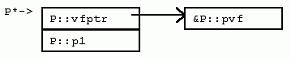1. struct  P {
2.    int  p1;
3.    void  pf();  // new
4.    virtual   void  pvf();  // new
5. };

[cpp] view plain
1. struct P {
2.    int p1;
3.    void pf(); // new
4.    virtual void pvf(); // new
5. };

P有一个非虚成员函数pf()，以及一个虚成员函数pvf()。很明显，虚成员 函数造成对象实例占用更多内存空间，因为虚成员函数需要虚函数表指针。这一点以后还会谈到。这里要特别指出的是，声明非虚成员函数不会造成任何对象实例的 内存开销。现在，考虑P::pf()的定义。

1. void  P::pf() {  // void P::pf([P *const this])
2.    ++p1;   // ++(this->p1);
3. }

[cpp] view plain
1. void P::pf() { // void P::pf([P *const this])
2.    ++p1;   // ++(this->p1);
3. }

5.1 覆盖成员函数

Q从P继承了成员变量和成员函数。Q声明了pf()，覆盖了P::pf()。Q还声明了pvf()，覆盖了P::pvf()虚函数。Q还声明了新的非虚成员函数qf()，以及新的虚成员函数qvf()。1. struct  Q : P {
2.    int  q1;
3.    void  pf();  // overrides P::pf
4.    void  qf();  // new
5.    void  pvf();  // overrides P::pvf
6.    virtual   void  qvf();  // new
7. };

[cpp] view plain
1. struct Q : P {
2.    int q1;
3.    void pf(); // overrides P::pf
4.    void qf(); // new
5.    void pvf(); // overrides P::pvf
6.    virtual void qvf(); // new
7. };

1. P p; P* pp = &p; Q q; P* ppq = &q; Q* pq = &q;
2. pp->pf(); // pp->P::pf(); // P::pf(pp);
3. ppq->pf(); // ppq->P::pf(); // P::pf(ppq);
4. pq->pf(); // pq->Q::pf(); // Q::pf((P*)pq); （错误!）
5. pq->qf(); // pq->Q::qf(); // Q::qf(pq);

[cpp] view plain
1. P p; P* pp = &p; Q q; P* ppq = &q; Q* pq = &q;
2. pp->pf(); // pp->P::pf(); // P::pf(pp);
3. ppq->pf(); // ppq->P::pf(); // P::pf(ppq);
4. pq->pf(); // pq->Q::pf(); // Q::pf((P*)pq); （错误!）
5. pq->qf(); // pq->Q::qf(); // Q::qf(pq);

1. pp->pvf();  // pp->P::pvf(); // P::pvf(pp);
2. ppq->pvf(); // ppq->Q::pvf(); // Q::pvf((Q*)ppq);
3. pq->pvf(); // pq->Q::pvf(); // Q::pvf((P*)pq); （错误！）

[cpp] view plain
1. pp->pvf(); // pp->P::pvf(); // P::pvf(pp);
2. ppq->pvf(); // ppq->Q::pvf(); // Q::pvf((Q*)ppq);
3. pq->pvf(); // pq->Q::pvf(); // Q::pvf((P*)pq); （错误！）

1. class  CA
2. {   int  a;};
3. class  CB
4. {   int  b;};
5. class  CL :  public  CB,  public  CA
6. {   int  c;};

[cpp] view plain
1. class CA
2. {   int a;};
3. class CB
4. {   int b;};
5. class CL : public CB, public CA
6. {   int c;};

int b;
int a;
int c;

1. class  CA
2. {
3.    int  a;
4.    virtual   void  seta(  int  _a ) { a = _a; }
5. };

[cpp] view plain
1. class CA
2. {
3.    int a;
4.    virtual void seta( int _a ) { a = _a; }
5. };

vfptr;
int a;
int b;
int c;

5.2 多重继承下的虚函数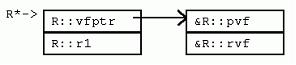1. struct  R {
2.    int  r1;
3.    virtual   void  pvf();  // new
4.    virtual   void  rvf();  // new
5. };
[cpp] view plain
1. struct R {
2.    int r1;
3.    virtual void pvf(); // new
4.    virtual void rvf(); // new
5. };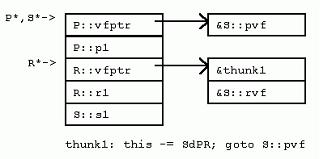1. struct  S : P, R {
2.    int  s1;
3.    void  pvf();  // overrides P::pvf and R::pvf
4.    void  rvf();  // overrides R::rvf
5.    void  svf();  // new
6. };
[cpp] view plain
1. struct S : P, R {
2.    int s1;
3.    void pvf(); // overrides P::pvf and R::pvf
4.    void rvf(); // overrides R::rvf
5.    void svf(); // new
6. };

1. S s; S* ps = &s;
2. ((P*)ps)->pvf(); // (*(P*)ps)->P::vfptr)((S*)(P*)ps)
3. ((R*)ps)->pvf(); // (*(R*)ps)->R::vfptr)((S*)(R*)ps)
4. ps->pvf();       // one of the above; calls S::pvf()
[cpp] view plain
1. S s; S* ps = &s;
2. ((P*)ps)->pvf(); // (*(P*)ps)->P::vfptr)((S*)(P*)ps)
3. ((R*)ps)->pvf(); // (*(R*)ps)->R::vfptr)((S*)(R*)ps)
4. ps->pvf();       // one of the above; calls S::pvf()

 调用((P*)ps)->pvf()时，先到P的虚函数表中取出第一项，然后把ps转化为S*作为this指针传递进去；
 调用((R*)ps)->pvf()时，先到R的虚函数表中取出第一项，然后把ps转化为S*作为this指针传递进去；

5.3 地址点与“逻辑this调整”

1. ((R*)ps)->rvf();  // (*((R*)ps)->R::vfptr)((R*)ps)

[cpp] view plain
1. ((R*)ps)->rvf(); // (*((R*)ps)->R::vfptr)((R*)ps)

1. ps->rvf();  // ((R*)ps)->rvf(); // S::rvf((R*)ps)

[cpp] view plain
1. ps->rvf(); // ((R*)ps)->rvf(); // S::rvf((R*)ps)

5.4 调整块

1. ps->rvf();
2. // struct { void (*pfn)(void*); size_t disp; };
3. // (*ps->vfptr[i].pfn)(ps + ps->vfptr[i].disp);

[cpp] view plain
1. ps->rvf();
2. // struct { void (*pfn)(void*); size_t disp; };
3. // (*ps->vfptr[i].pfn)(ps + ps->vfptr[i].disp);

2. this  -= SdPR;
3. goto  S::pvf()

[cpp] view plain
2. this -= SdPR;
3. goto S::pvf()

2. this  -= SdPR;  // fall into S::pvf()
3. S::pvf() { ... }

[cpp] view plain
2. this -= SdPR; // fall into S::pvf()
3. S::pvf() { ... }

5.5 虚继承下的虚函数

T虚继承P，覆盖P的虚成员函数，声明了新的虚函数。如果采用在基类虚函数表末尾添加新项的方式，则访问虚函数总要求访问虚基类。在VC++中，为了避免获取虚函数表时，转换到虚基类P的高昂代价，T中的新虚函数通过一个新的虚函数表获取 ，从而带来了一个新的虚函数表指针。该指针放在T实例的顶端。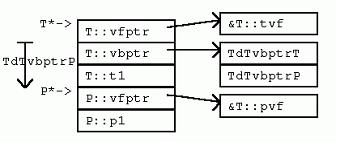1. struct  T :  virtual  P {
2.    int  t1;
3.    void  pvf();          // overrides P::pvf
4.    virtual   void  tvf();  // new
5. };
6. void  T::pvf() {
7.    ++p1; // ((P*)this)->p1++; // vbtable lookup!
8.    ++t1; // this->t1++;
9. }

[cpp] view plain
1. struct T : virtual P {
2.    int t1;
3.    void pvf();         // overrides P::pvf
4.    virtual void tvf(); // new
5. };
6. void T::pvf() {
7.    ++p1; // ((P*)this)->p1++; // vbtable lookup!
8.    ++t1; // this->t1++;
9. }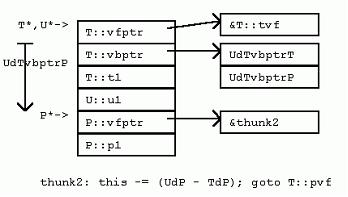1. struct  U : T {
2.    int  u1;
3. };

[cpp] view plain
1. struct U : T {
2.    int u1;
3. };

5.6 特殊成员函数

5.6.1 构造函数和析构函数

1 * 如果是“最终派生类”，初始化vbptr成员变量，调用虚基类的构造函数；
2 * 调用非虚基类的构造函数
3 * 调用成员变量的构造函数
4 * 初始化虚函数表成员变量
5 * 执行构造函数体中，程序所定义的其他初始化代码

（注意：一个“最终派生类”的实例，一定不是嵌套在其他派生类实例中的基类实例）

1 * 合成并初始化虚函数表成员变量
2 * 执行析构函数体中，程序定义的其他析构代码
3 * 调用成员变量的析构函数（按照相反的顺序）
4 * 调用直接非虚基类的析构函数（按照相反的顺序）
5 * 如果是“最终派生类”，调用虚基类的析构函数（按照相反顺序）

5.6.2 虚析构函数与delete操作符

A* p = new B();

delete (B*)p;

delete p;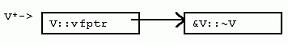1. struct  V {
2.    virtual  ~V();
3. };
[cpp] view plain
1. struct V {
2.    virtual ~V();
3. };1. struct  W : V {
2.    operator delete ();
3. };
[cpp] view plain
1. struct W : V {
2.    operator delete();
3. };

1. V* pv =  new  V;
2. delete  pv;    // pv->~V::V(); // use ::operator delete()
3. pv = new  W;
4. delete  pv;    // pv->~W::W(); // use W::operator delete() 动态绑定到 W的析构函数，W默认的析构函数调用{delete this;}
5. pv = new  W;
6. ::delete  pv;  // pv->~W::W(); // use ::operator delete()
[cpp] view plain
1. V* pv = new V;
2. delete pv;   // pv->~V::V(); // use ::operator delete()
3. pv = new W;
4. delete pv;   // pv->~W::W(); // use W::operator delete() 动态绑定到 W的析构函数，W默认的析构函数调用{delete this;}
5. pv = new W;
6. ::delete pv; // pv->~W::W(); // use ::operator delete()

 V没有定义delete操作符，delete时使用函数库的delete操作符；
 W定义了delete操作符，delete时使用自己的delete操作符；
 可以用全局范围标示符显示地调用函数库的delete操作符。

6 数组

1、 堆上分配空间的数组，由于数组可大可小，所以，数组大小值应该和数组一起保存。因此，堆上分配空间的数组会分配额外的空间来存储数组元素的个数；
2、 当数组被删除时，数组中每个元素都要被正确地释放，即使当数组大小不确定时也必须成功完成该操作。然而，派生类可能比基类占用更多的内存空间，从而使正确释放比较困难。

1. struct  WW : W {  int  w1; };
2. pv = new  W[m];
3. delete  [] pv;  // delete m W's (sizeof(W) == sizeof(V))
4. pv = new  WW[n];
5. delete  [] pv;  // delete n WW's (sizeof(WW) > sizeof(V))
[cpp] view plain
1. struct WW : W { int w1; };
2. pv = new W[m];
3. delete [] pv; // delete m W's (sizeof(W) == sizeof(V))
4. pv = new WW[n];
5. delete [] pv; // delete n WW's (sizeof(WW) > sizeof(V))

7 异常处理

1. struct  X { X(); };  // exception object class
2. struct  Z { Z(); ~Z(); };  // class with a destructor
3. extern   void  recover( const  X&);
4. void  f( int ), g( int );
5.
6. int  main() {
7.    try  {
8.       f(0);
9.    } catch  ( const  X& rx) {
10.       recover(rx);
11.    }
12.    return  0;
13. }
14.
15. void  f( int  i) {
16.    Z z1;
17.    g(i);
18.    Z z2;
19.    g(i-1);
20. }
21.
22. void  g( int  j) {
23.    if  (j < 0)
24.       throw  X();
25. }
[cpp] view plain
1. struct X { X(); }; // exception object class
2. struct Z { Z(); ~Z(); }; // class with a destructor
3. extern void recover(const X&);
4. void f(int), g(int);
5. int main() {
6.    try {
7.       f(0);
8.    } catch (const X& rx) {
9.       recover(rx);
10.    }
11.    return 0;
12. }
13. void f(int i) {
14.    Z z1;
15.    g(i);
16.    Z z2;
17.    g(i-1);
18. }
19. void g(int j) {
20.    if (j < 0)
21.       throw X();
22. }

8 小结C++类和对象【3】—— C++对象模型和this指针（const修饰的常函数与常对象）
C++类和对象【3】—— C++对象模型和this指针（const修饰的常函数与常对象）
0 0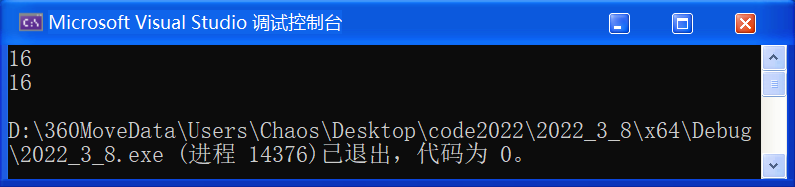【C++要笑着学】类和对象 | 初识封装 | 访问限定符 | 类的作用域和实例化 | 类对象模型 | this指针（二）

0 0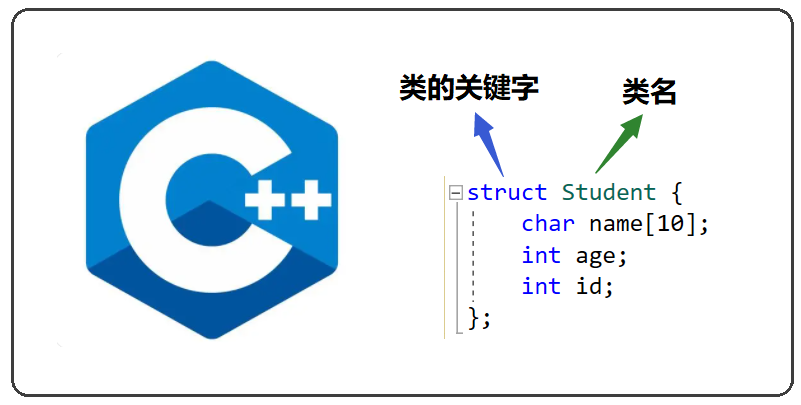【C++要笑着学】类和对象 | 初识封装 | 访问限定符 | 类的作用域和实例化 | 类对象模型 | this指针（一）

0 0C++对象模型分析
C++对象模型分析
0 0C++继承中的对象模型与继承中构造和析构顺序

0 0C++对象模型和this指针实例分析（二）

0 0C++对象模型和this指针实例分析

0 0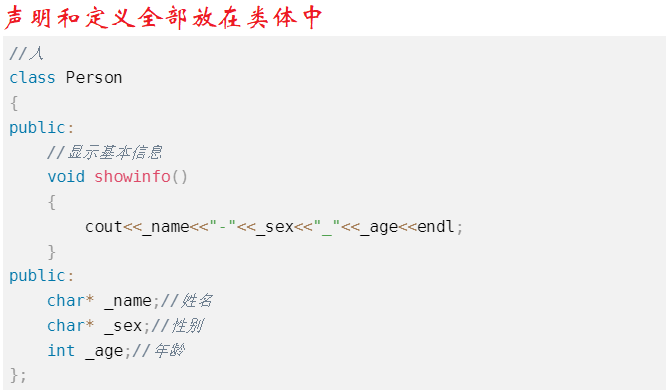【C++初阶：类和对象(上篇)】类的定义 | 类的访问限定符及封装 | 类的作用域 | 类的实例化 | 类对象模型 | this指针
【C++初阶：类和对象(上篇)】类的定义 | 类的访问限定符及封装 | 类的作用域 | 类的实例化 | 类对象模型 | this指针
0 00 02275 0
redrain.

C++课程-对象模型

C++对象模型

C++对象模型